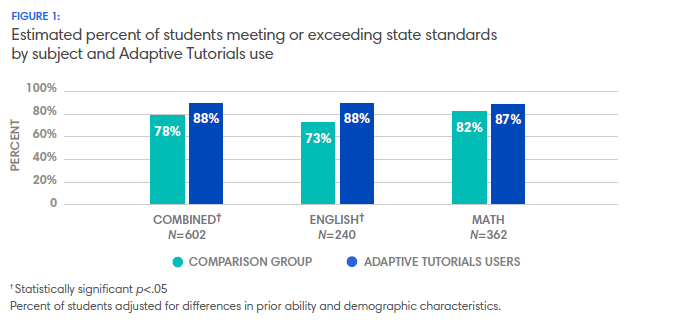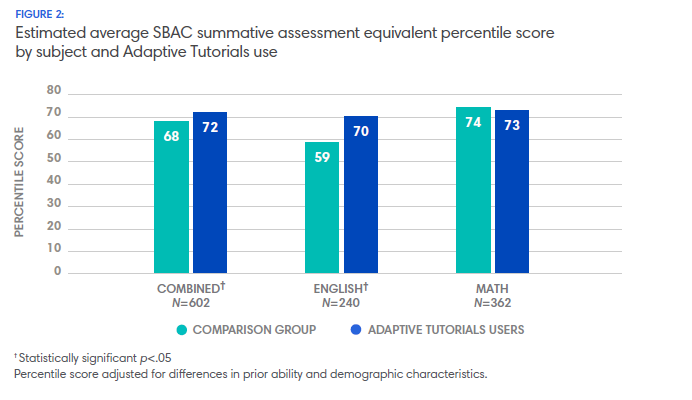ESSA Level: moderate

# A Study of the Efficacy of Apex Learning Adaptive Tutorials on Middle School Student Achievement Year 1

April 13, 2017
Beaverton School District, OR
delimiter

Do middle school students using Apex Learning Adaptive Tutorials have the same or better chances of meeting or exceeding the state standards (Level 3 or above) on the SBAC summative assessments as similar students not using Adaptive Tutorials?

Overall, students using Adaptive Tutorials were 13% more likely (.88 / .78) to meet or exceed the state standard on the SBAC assessments than students participating in classroom instruction alone (OR=2.06, p<.03, ES=.44). By subject, students using Tutorials were 20% more likely (.88 / .73) to meet or exceed the state standard for English language arts (OR=2.73, p<.03, ES=.61) and 6% more likely (.87 / .82) for mathematics (OR=1.48, p<.10, ES=.24) than similar students participating in traditional classroom instruction alone. Table 3 in the appendix shows the number of enrollments in each group meeting or exceeding the standard and Table 4 shows the probability, odds, and risk ratio for meeting or exceeding the state standards by Tutorials use on the assessments overall and by subject.

Figure 1 shows the percent of students meeting or exceeding the standard by Adaptive Tutorials use and subject. The percent of students meeting or exceeding the standard on assessments overall was 10 percentage points greater (.88 - .78) for those using Tutorials than similar students not using Tutorials (z=3.29, p<.00). By subject, the percent of students meeting or exceeding the standard on the English language arts assessments was 15 percentage points greater (.88 - .73) for students using Tutorials than students not using Tutorials (z=2.95, p<.00). The difference between the percent of students meeting or exceeding the standard for mathematics trended higher (.87 -. 82) for students using Tutorials than for students not using Tutorials and approached statistical significance (z=1.30, p<.10).Do middle school students using Apex Learning Adaptive Tutorials perform the same or better on SBAC summative assessments as similar students not using Adaptive Tutorials?

Overall, the average student using Adaptive Tutorials in addition to classroom instruction (M=.61, SD=.65) performed significantly better on the SBAC summative assessment than the average student participating in traditional classroom instruction alone (M=.49, SD=.94) (t(601)=2.99, p<.00, ES=.12).

Figure 2 shows the average equivalent percentile score for students using Tutorials and similar students participating in traditional classroom instruction alone. Students using Tutorials achieved an additional 4 percentile points on the SBAC summative assessments combined and an additional 11 percentile points on the English language arts assessments compared to similar students (t(601)=4.15, p<.00, ES=.30). Students using Tutorials for math achieved similar average scores on the mathematics assessments as students participating in traditional classroom instruction alone.Tags
Category